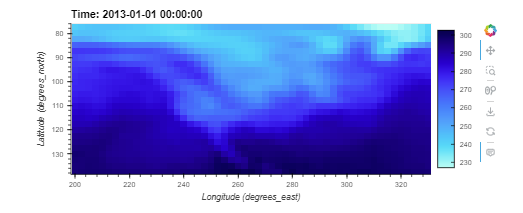# Hvplot image invert y axis

Hey folks,

I’m trying to invert y axis on hvplot.image() but not getting the result I thought would get. The data appears to be getting shifted and also does not appear to be inverted. Is this an issue that should be raised or have I missed something?

panel 1.1.0
hvplot 0.8.4
holoviews 1.16.2

``````import hvplot.xarray  # noqa
import xarray as xr
ds = xr.tutorial.open_dataset('air_temperature')
ds.hvplot.image()
``````

``````import hvplot.xarray  # noqa
import xarray as xr
ds = xr.tutorial.open_dataset('air_temperature')
ds.hvplot.image().opts(invert_yaxis=True)
``````

It appears data has been shifted rather than invertedThanks, Carl.

``````import hvplot.xarray  # noqa
import xarray as xr
ds = xr.tutorial.open_dataset('air_temperature')
ds.hvplot.image(ylim=(70, 20))
``````

Might be easier to set ylim backwards

However, for invert_y, can you submit an issue on holoviews?

1 Like
1 Like

Thanks for the alternative @ahuang11 appreciated.

Cheers

2 Likes